# S3E3 - How to Find the Empirical Formula and Molecular Formula

We'll do some empirical formula calculations and problems in just a second, but first let's get some definitions out of the way.

## Empirical Formula

Empirical Formula = the simplest whole-number ratio of atoms in a compound.

## Molecular Formula

Molecular Formula = the exact formula of a compound.

-----

Here's how the two formulas are related:

Molecular Formula  =  n ( Empirical Formula)
-- where  n = whole-number integer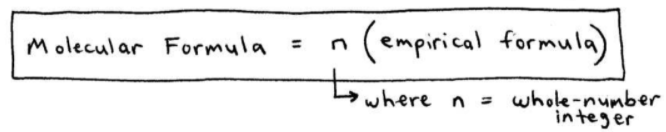=====

## Empirical Formula Calculations

Let's take a look at a few sample problems...

As always, we learn best by watching example calculations and using them to help us with our own problems and calculations.

ex:  Suppose a compound is 71.65% chlorine, 24.27% carbon, and 4.07% hydrogen.  It's molar mass is 98.96 g/mol.  Find the empirical and molecular formulas.
_________

➞  always find the empirical formula first. Always!
➞  even if you're only asked to find the molecular formula.

Step 1.  Assume you have 100g of material and convert to moles.

Step 2.  Divide by the lowest number of moles:

(71.65g Cl / 1) * (1 mol Cl / 35.45g Cl)  =  2.021 mol Cl  / 2.021  =  1 mol Cl

(24.27g C / 1) * (1 mol C / 12.01g C)  =  2.021 mol C  /  2.021  =  1 mol C

(4.07 g H / 1) * (1mol H / 1.01g H)  =  4.04 mol H  /  2.021  =  2 mol H

Here that is again, in a more relaxing easier-to-follow handwritten format: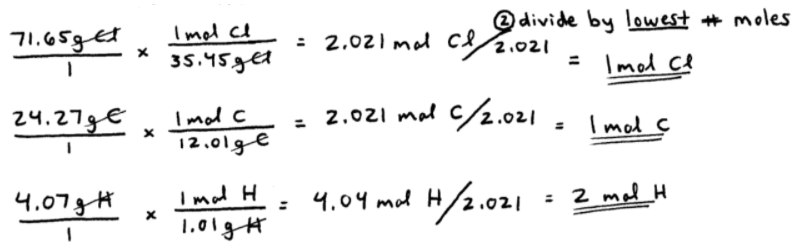-----

Step 3.  Combine the moles of each atom into an empirical formula:

CH2Cl  =  empirical formula.  Carbon (C) is always first if all elements are nonmetals.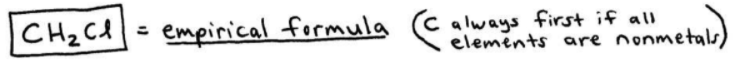Since we were asked to also find the molecular formula, two additional steps are needed...

-----

Step 4.  Calculate the empirical formula mass.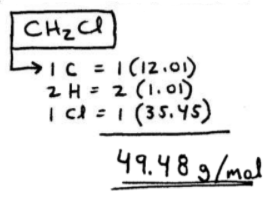-----

Step 5.  Plug the empirical mass and the molar mass into the boxed-up formula from earlier in this post:

Molecular Formula  =  n ( Empirical Formula)
-- where  n = whole-number integer

98.96 g/mol  =  n ( 49.48g/mol)

n = 2  ➞  2  [ CH2Cl ]  =  C2H4Cl2  ➞  this is the molecular formula.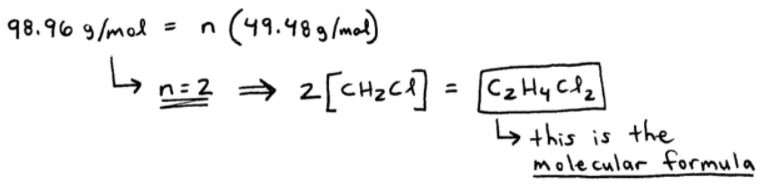=====

ex:  A compound containing only nitrogen and oxygen is 30.4% nitrogen by mass. If the molar mass of the compound is 92 g/mol, what's the molecular formula?
_________

➞  always find the empirical formula first. Always!
➞  even if you're only asked to find the molecular formula.

Step 1.  Assume 100g, so we have 30.4g N and 69.6g O.  Convert to moles.

Step 2.  Divide by the lowest number of moles.

Step 3.  Combine the moles of each atom into an empirical formula:

(30.4g N / 1) * (1 mol N / 14.01g N)  =  2.17 mol N  /  2.17  =  1 mol N

(69.6g O / 1) * (1 mol O / 16.00g O)  =  4.35 mol O  /  2.17  =  2 mol O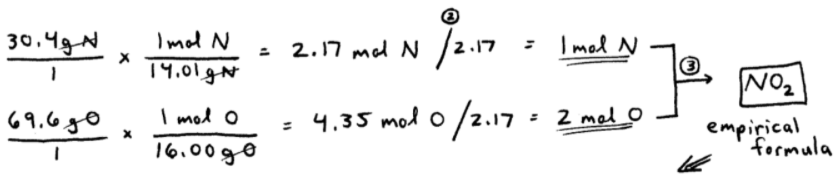-----

Step 4.  Calculate the empirical formula mass.

Step 5.  Plug the empirical mass and the molar mass into the boxed-up formula from earlier in this post:

92 g/mol  =  n ( 46.01g/mol)

n = 2  ➞  2  [ NO2 ]  =  N2O4  ➞  this is the molecular formula.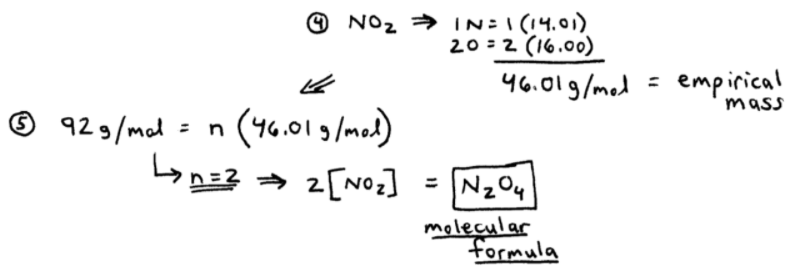=====

QUESTION:  What would you do if after STEP 2 above, you had 1.33 mol N and 2 mol O ?
_________

Well... 1.33mol N  =  1 1/3 mol N  =  4/3 mol N

So we have:

4/3 mol N  (x 3)  =  4 mol N
2 mol O  (x 3)  =  6 mol O

➞  N4O6  ➞  our "new" empirical formula.-----

Now, besides the "1.33 mol N" issue that can occur, also watch out for "1.67 moles" and "1.5 moles" situations.  See here: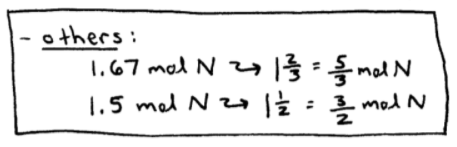=====

That's it for my 3rd post covering SECTION 3 - Chemical Quantities and Stoichiometry

The next topics in this Section ("Chapter") include:

Balancing Chemical Reactions and Reaction Stoichiometry: Moles-to-Moles, Mass-to-Moles, Moles-to-Mass, and Mass-to-Mass Calculations.